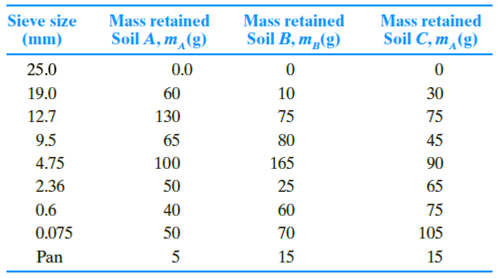Chapter 2, Problem 2.2CTPPrinciples of Geotechnical Enginee...

9th Edition
Braja M. Das + 1 other
ISBN: 9781305970939

Solutions

Chapter
SectionPrinciples of Geotechnical Enginee...

9th Edition
Braja M. Das + 1 other
ISBN: 9781305970939
Textbook Problem

Refer to Problem 2.C.1. Results of the sieve analysis for Soils A, B, and C are given below. To obtain a more representative sample for further geotechnical testing, a ternary blend is created by uniformly mixing 8000 kg of each soil. Answer the following questions.a. If a sieve analysis is conducted on the mixture using the same set of sieves as shown above, compute the mass retained (as a percentage) and cumulative percent passing in each sieve.b. What would be the uniformity coefficient (Cu) and the coefficient of gradation (Cc) of the mixture?

(a)

To determine

Calculate the mass retained and cumulative percent passing in each sieve.

Explanation

Given information:

The sieve analysis for soils A, B, and C are given in the Table.

Mass of each soil is 8,000kg.

Calculation:

Calculate the total mass of the ternary blend as shown below.

Total mass in the ternary mix=3×8,000=24,000kg

Calculate the percent of each soil in the mix as shown below.

Percent of each soil=Mass of each soilTotal mass in the ternary mix×100

Substitute 8,000kg for mass of each soil and 24,000kg for total mass of the ternary mix.

Percent of each soil(A,B,C)=8,00024,000×100=33.33%

Calculate the total mass of the soil (M) using the relation.

M=M1+M2++Mn+Mp

Here, M1+M2++Mn is mass of soil retain in corresponding sieve number and Mp is the mass of soil retained in the pan.

Refer the given table for mass of soil retained in each sieve no.

Calculate the total mass of the soil A as follows:

mA=0+60+130+65+100+50+40+50+5=500g

Calculate the total mass of the soil B as follows:

mB=0+10+75+80+165+25+60+70+15=500g

Calculate the total mass of the soil C as follows:

mC=0+30+75+45+90+65+75+105+15=500g

Calculate the percent of mass retained on each sieve for the ternary mix as shown below.

mM=[%ofsoilA(mAmA×100)+%ofsoilB(mBmB×100)+%ofsoilA(mCmC×100)]

Substitute 33.33% for %ofsoilA, 33

(b)

To determine

Calculate the uniformity coefficient and coefficient of gradation of the mixture.

Still sussing out bartleby?

Check out a sample textbook solution.

See a sample solution

The Solution to Your Study Problems

Bartleby provides explanations to thousands of textbook problems written by our experts, many with advanced degrees!

Get Started

List and describe the different types of databases.

Database Systems: Design, Implementation, & Management

How is a ring test performed?

Precision Machining Technology (MindTap Course List)

What are the cornerstones to an organizations security program?

Principles of Information Systems (MindTap Course List)

Determine the axial force in member BC of the frame. Note the joint D is a sliding pin.

International Edition---engineering Mechanics: Statics, 4th Edition